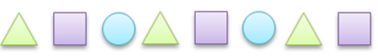Mathematics
Easy

Question

# Which shape will come next in the repeating pattern?The rule is "Triangle, Square, Circle”:## Square    Triangle    Rectangle    CircleHint:

## The correct answer is: Circle

### The given pattern in words is Triangle, Square, Circle, Triangle, Square, Circle, Triangle, SquareWe are asked to find the next shape.The rule used to write the pattern is “ Triangle, Square and Circle.”Three shapes are repeating.The last shape is a square. And according to the rule, the shape that will come after the square is a circle.Therefore, the next shape is a circle.

While writing the pattern, we should be careful about the sequence of the elements.

### Related Questions to study#### With Turito Foundation.#### Get an Expert Advice From Turito.